# Financial Volatility Modelling¶

There are some time series where the variance changes consistently over time. In the context of financial time series, this would be called increasing or decreasing volatility.

In time series where the variance is increasing in a systematic way, such as an increasing trend, this property of the series is called heteroskedasticity. If the change in variance can be correlated over time, then it can be modeled using an autoregressive process, such as an ARCH.

Autoregressive Conditional Heteroskedasticity (ARCH) models explicitly allow modelling of the change in variance over time in a time series, something that classical ARMA models do not allow.

In this section we will present an example of esimating ARCH and GARCH models with Python. We will use the arch_model function from the arch package.

To install the package, follow the documentation - open your command prompt (as long as you selected to add anaconda to your PATH during the installation) and enter the following:

conda install -c bashtage arch

If you do not have anaconda, use pip install arch

Note that this package works on a 64-bit OS only.

In :
#Import the required modules for vectors and matrix operations, data generation
import numpy as np
#Import the required modules for plot creation:
import matplotlib.pyplot as plt
#import the required modules for TimeSeries data generation:
import statsmodels.api as sm
#Import the required modules for test statistic calculation:
import statsmodels.stats as sm_stat
#Import the required modules for time series model estimation:
import statsmodels.tsa as smt
#Import Pandas
import pandas as pd

In :
import arch as arch


you can read the documentation on arch with

In :
arch.doc()


Other usefull functions:

In :
def tsdisplay(y, figsize = (14, 8), title = "", lags = 20):
tmp_data = pd.Series(y)
fig = plt.figure(figsize = figsize)
#Plot the time series
tmp_data.plot(ax = fig.add_subplot(311), title = "$Time\ Series\ " + title + "$", legend = False)
#Plot the ACF:
sm.graphics.tsa.plot_acf(tmp_data, lags = lags, zero = False, ax = fig.add_subplot(323))
plt.xticks(np.arange(1,  lags + 1, 1.0))
#Plot the PACF:
sm.graphics.tsa.plot_pacf(tmp_data, lags = lags, zero = False, ax = fig.add_subplot(324))
plt.xticks(np.arange(1,  lags + 1, 1.0))
#Plot the QQ plot of the data:
plt.title("QQ Plot")
#Plot the residual histogram:
fig.add_subplot(326).hist(tmp_data, bins = 40, normed = 1)
plt.title("Histogram")
#Fix the layout of the plots:
plt.tight_layout()
plt.show()

In :
def tsdiag(y, figsize = (14,8), title = "", lags = 10):
#The data:
tmp_data = pd.Series(y)
#The Ljung-Box test results for the first k lags:
tmp_acor = list(sm_stat.diagnostic.acorr_ljungbox(tmp_data, lags = lags, boxpierce = True))
# get the p-values
p_vals = pd.Series(tmp_acor)
#Start the index from 1 instead of 0 (because Ljung-Box test is for lag values from 1 to k)
p_vals.index += 1
fig = plt.figure(figsize = figsize)
#Plot the p-values:
p_vals.plot(ax = fig.add_subplot(313), linestyle='', marker='o', title = "p-values for Ljung-Box statistic", legend = False)
#Add the horizontal 0.05 critical value line
plt.axhline(y = 0.05, color = 'blue', linestyle='--')
# Annotate the p-value points above and to the left of the vertex
x = np.arange(p_vals.size) + 1
for X, Y, Z in zip(x, p_vals, p_vals):
plt.annotate(round(Z, 4), xy=(X,Y), xytext=(-5, 5), ha = 'left', textcoords='offset points')
plt.show()
# Return the statistics:
col_index = ["Ljung-Box: X-squared", "Ljung-Box: p-value", "Box-Pierce: X-squared", "Box-Pierce: p-value"]
return pd.DataFrame(tmp_acor, index = col_index, columns = range(1, len(tmp_acor) + 1))

In :
# Suppress matplotlib's annoying deprecation warning
import warnings
import matplotlib.cbook
warnings.filterwarnings("ignore", category = matplotlib.cbook.mplDeprecation)


## ARCH: General Idea and Simulation¶

We can think of $\rm ARCH$ models as $\rm AR$ model analogue applied to the error variance of a time series $r_t$ (where $r_t$ are usually. More specifically, it models the variance at time $t$ as a function of the residual errors from a mean process.

For example, we define the following $\rm ARCH(1)$ process:

$\begin{cases} r_t &= \mu + \epsilon_t \\ \epsilon_t &= \sigma_t w_t,\quad w_t \sim WN(0, \sigma^2)\\ \sigma_t^2 &= \omega + \alpha_1 \epsilon^2_{t-1} \end{cases}$

In general, a volatility modelling includes the following components:

• $\mu$ - the mean model. This can be considered the first component in volatility model building. Examples include:

• zero mean: $r_t = \epsilon_t$;
• constant mean: $r_t = \mu + \epsilon_t$;
• an $\rm AR(p)$ with constant: $r_t = \gamma + \sum_{j=1}^p \phi_{j} r_{t-j} + \epsilon_t$
• more complex models, like an $\rm ARMA(p,q)$ process with exogeneous variables and so on.
• $\sigma_t^2 = ...$ - a volatility model, which is added to the mean model to capture time-varying volatility. In addition to $\rm ARCH$, other specifications are available, like: the constant variance, $\rm GARCH$, $\rm EGARCH$, $\rm IGARCH$, $\rm GJR-GARCH$, $\rm TGARCH$ and more.

• $w_t \sim WN(0, \sigma^2)$ - the distribution of $w_t$ is the final component of a volatility model. Examples include - standard normal, Student t, GED (Generalized Error Distribution) and so on.

We will simulate an $\rm ARCH(1)$ process as follows:

In :
np.random.seed(123)
#Define the sample size:
n = 2000
# Define the coefficients:
mu = 1.0
omega = 2.0
alpha = 0.5
# Generate the random shocks
w = np.random.normal(size = n)
#
# Generate the ARCH process:
r_t = np.array(mu + np.sqrt(omega) * w, ndmin = 1)
for j in range(1, n):
r_t = np.append(r_t, mu + np.sqrt(omega + alpha * ((r_t[j-1] - mu)**2)) * w[j])


We can examine the series:

In :
tsdisplay(r_t)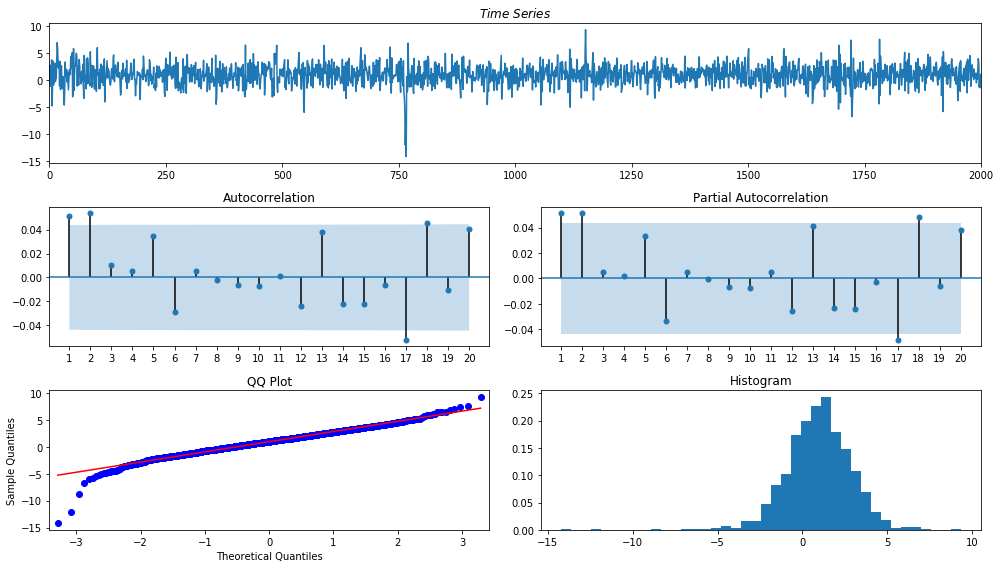In :
tsdiag(r_t)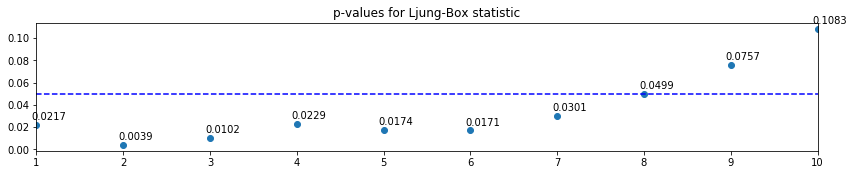Out:
1 2 3 4 5 6 7 8 9 10
Ljung-Box: X-squared 5.266093 11.073378 11.292001 11.348124 13.728753 15.441068 15.502509 15.513726 15.599786 15.708086
Ljung-Box: p-value 0.021745 0.003940 0.010247 0.022918 0.017427 0.017090 0.030071 0.049893 0.075724 0.108299
Box-Pierce: X-squared 5.258201 11.053884 11.271961 11.327915 13.700221 15.405694 15.466858 15.478019 15.563606 15.671257
Box-Pierce: p-value 0.021844 0.003978 0.010343 0.023116 0.017630 0.017325 0.030459 0.050491 0.076573 0.109438

The first lags appear to be correlated. Now let's plot the squared time series:

In :
tsdisplay(r_t**2)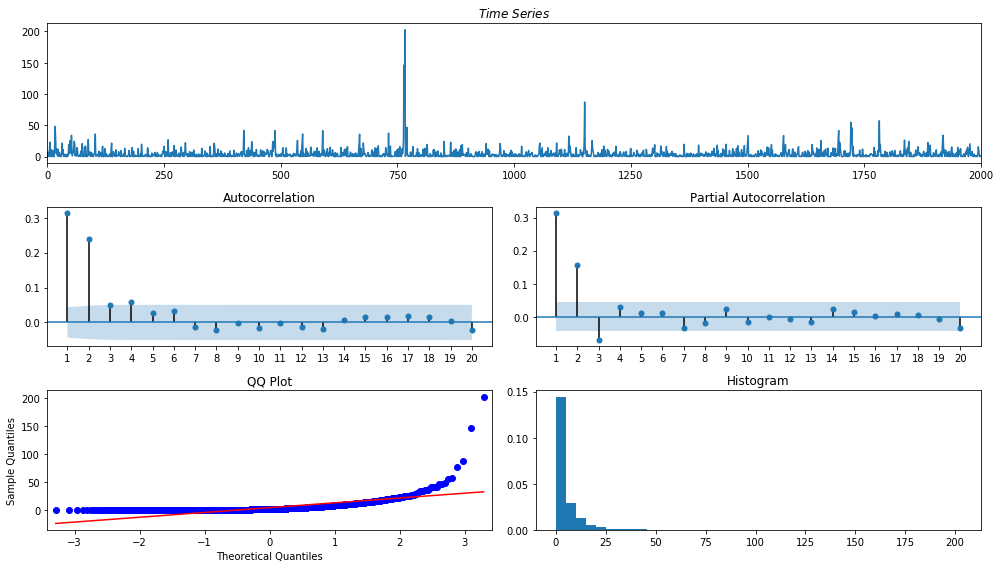We see a strong autocorrelation, which may indicate the possibility of ARCH effects.

If we wanted to estimate an ARCH model on this data, we would first have to create a mean model for the returns r_t. Then we should inspect the residuals - if their ACF and PACF plots show no autocorrelation but the squared residual ACF and PACF plots - show autocorrelation - we should create an ARCH model for the residuals.

We leave this as an exercise and instead opt to show the detailed modelling steps for the more general, $\rm GARCH$ model. These same steps can also be applied to the $\rm ARCH$ model specification.

## GARCH: simulation and model building example¶

A $\rm GARCH$ model can be thought of an an ARMA model analogue applied to the error variance of a time series.

We will consider the following $\rm GARCH(1, 1)$ example:

$\begin{cases} r_t &= \mu + \epsilon_t \\ \epsilon_t &= \sigma_t w_t \\ \sigma_t^2 &= \omega + \alpha_1 \epsilon^2_{t-1} + \beta_1 \sigma^2_{t-1} \end{cases}$

where $\mu = 1$, $\omega = 0.2$, $\alpha = 0.5$ and $\beta = 0.3$ and $w_t \sim \mathcal{N}(0, 1)$.

In :
np.random.seed(1)
#Define the sample size:
n = 2000
# Define the coefficients:
mu = 1.0
omega = 0.2
alpha = 0.5
beta  = 0.3
# Generate the random shocks
w = np.random.normal(size = n)
sigma_sq = np.zeros_like(w)
#
# Generate the GARCH process:
sigma_sq = omega
r_t = np.array(mu + np.sqrt(sigma_sq) * w, ndmin = 1)
for j in range(1, n):
sigma_sq[j] = omega + alpha * ((r_t[j-1] - mu)**2 + beta * sigma_sq[j-1])
r_t = np.append(r_t, mu + np.sqrt(sigma_sq[j]) * w[j])


We will again examine the ACF's of the series:

In :
tsdisplay(r_t, title = "of\ r_t")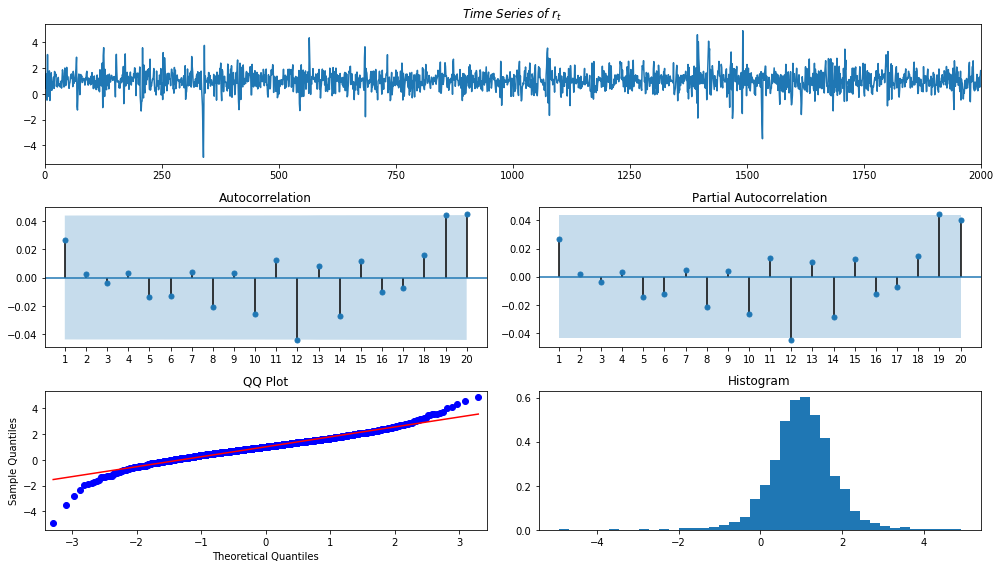If we test for significant autocorrelations

In :
tsdiag(r_t)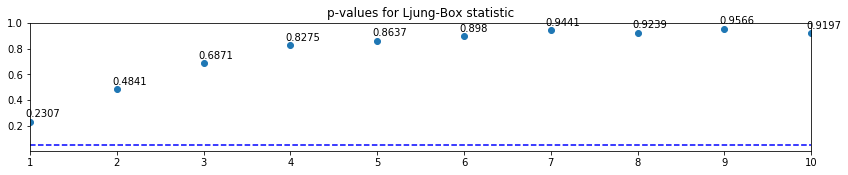Out:
1 2 3 4 5 6 7 8 9 10
Ljung-Box: X-squared 1.436600 1.450969 1.479100 1.495282 1.893443 2.223409 2.258945 3.160586 3.181938 4.539475
Ljung-Box: p-value 0.230690 0.484090 0.687102 0.827477 0.863684 0.898048 0.944130 0.923879 0.956643 0.919747
Box-Pierce: X-squared 1.434448 1.448787 1.476848 1.492982 1.889751 2.218398 2.253774 3.150912 3.172146 4.521547
Box-Pierce: p-value 0.231040 0.484618 0.687624 0.827884 0.864180 0.898557 0.944471 0.924533 0.957077 0.920768

It would appear that our series is $WN$.

However, we do note that if $\epsilon_t \sim WN$, then their squared values should not be autocorrelated as well. We will test this

In :
tsdisplay(r_t**2, title = "of\ r_t^2")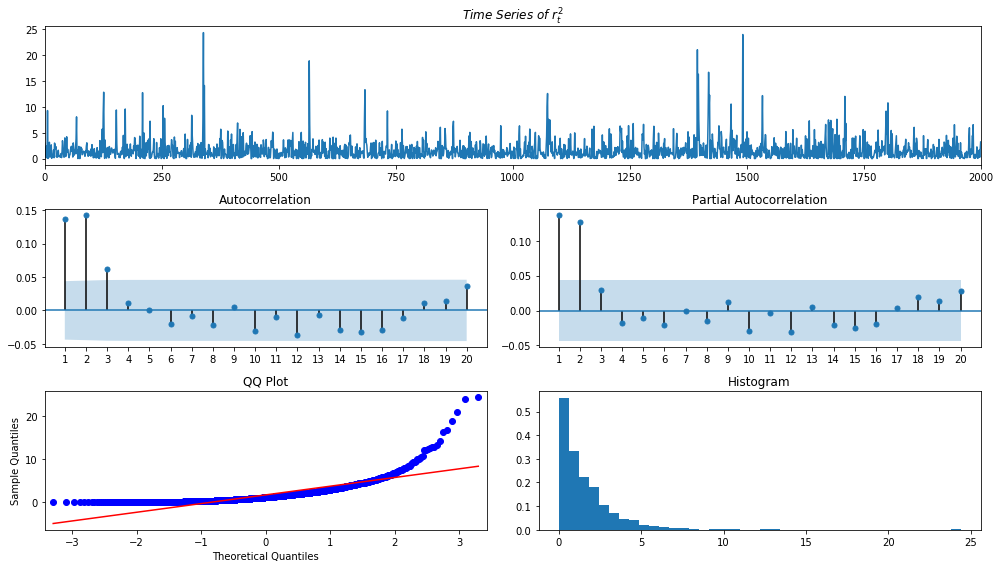We see that the autocorrelation is significant, which indicates ARCH effects.

In comparison, a simple WN (in this case, a standard normal) process does not have this property:

In :
tsdisplay(w**2, title = "w_t^2", figsize = (15, 8))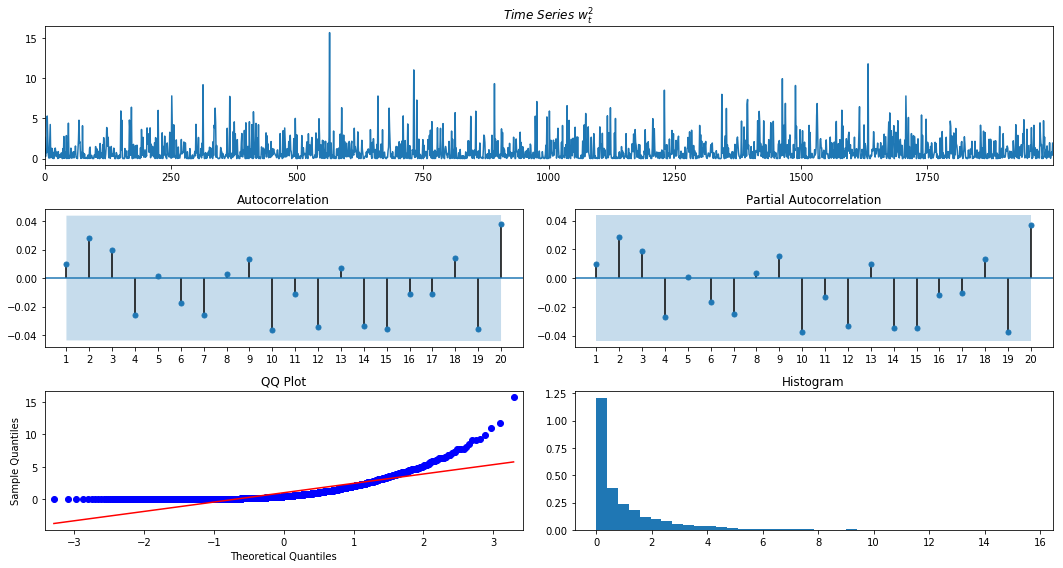We will supress additional warning messages to make it easier to read the output. These messages include:

• FutureWarning:

  "Using a non-tuple sequence for multidimensional indexing is deprecated; use arr[tuple(seq)] instead of arr[seq].
In the future this will be interpreted as an array index, arr[np.array(seq)], which will result either in an error or a different result."


This is a warning specific to statsmodels estimation, which will hopefully be adressed in a package update.

• RuntimeWarning:

  "Invalid value encountered in true_divide newparams = ((1-np.exp(-params))/(1+np.exp(-params))).copy()"


This is again a warning in statsmodels for some lag order combinations. Again, the hope is that it will be adressed in the future.

• HessianInversionWarning:

  "Inverting hessian failed, no bse or cov_params available"


This is again a warning in statsmodels for some lag order combinations.

• ConvergenceWarning:

  "Maximum Likelihood optimization failed to converge. Check mle_retvals"


This is again a warning in statsmodels for some lag order combinations, which do not lead ot a convergence in the maximum likelihood function.

In :
warnings.filterwarnings("ignore", category = FutureWarning)
warnings.filterwarnings("ignore", category = RuntimeWarning)
warnings.filterwarnings("ignore", category = sm.tools.sm_exceptions.HessianInversionWarning)
warnings.filterwarnings("ignore", category = sm.tools.sm_exceptions.ConvergenceWarning)


### (1) Specifying a mean model¶

We begin by creating the model for the mean by looping through all possible $\rm ARMA(p, q)$ model combinations with $p_\max=q_\max=1$.

In :
# Specify the best AIC - default is infinite - so anything lower is better
best_aic = np.inf
# Specify the best order
best_order = None
# Specify the best model
best_mdl = None
# Loop through different (p, q) combinations, starting from ARMA(0, 0), to ARMA(1, 1)
pq_rng = range(2) # range(5) would be [0,1,2,3,4]
for i in pq_rng:
for j in pq_rng:
try:
#print("Fitting an ARMA(" + str(i), ", " + str(j) + ") model")
tmp_mdl = smt.api.ARIMA(r_t, order = (i,0,j)).fit(method = 'mle', trend = 'c')
tmp_aic = tmp_mdl.aic
if tmp_aic < best_aic:
best_aic = tmp_aic
best_order = (i, 0, j)
best_mdl = tmp_mdl
print("Fitted an ARMA(" + str(i), ", " + str(j) + ") model - it was better")
else:
print("Fitted an ARMA(" + str(i), ", " + str(j) + ") model - it was worse")
except: continue

Fitted an ARMA(0 , 0) model - it was better
Fitted an ARMA(0 , 1) model - it was worse
Fitted an ARMA(1 , 0) model - it was worse
Fitted an ARMA(1 , 1) model - it was worse

In :
best_aic

Out:
4648.563915165161
In :
print(best_mdl.summary().tables)

==============================================================================
coef    std err          z      P>|z|      [0.025      0.975]
------------------------------------------------------------------------------
const          1.0193      0.017     58.988      0.000       0.985       1.053
==============================================================================


We could have used an already established function to automatically select the order:

In :
mdl_auto = sm.tsa.stattools.arma_order_select_ic(r_t, ic = 'bic', trend = 'c', max_ar = 4, max_ma = 4)

In :
print(mdl_auto)

{'bic':              0            1            2            3            4
0  4659.765720  4665.937976  4673.522338  4681.092451  4688.665073
1  4665.931050  4673.525965  4681.087045  4688.655576  4696.218524
2  4673.524263  4680.685774  4684.166055          NaN  4698.189203
3  4681.094948  4688.047263  4693.808986  4699.887352  4703.541366
4  4688.677420  4693.262785  4698.164081  4703.602001  4707.921521, 'bic_min_order': (0, 0)}

In :
print(mdl_auto.bic_min_order)

(0, 0)


We chose our manual specification to highlight that we can always specify our own automated procedures. They may not be exactly as the ones readily provided in the packages, but we may not always need very generalized methods.

Note that sometimes having common roots results in NaN values in estimated parameters link.

As we have seen from the ACF and PACF plot of $r_t$, there is no need to create an $\rm ARMA$ model for the mean model and we got the same results from our $\rm ARMA$ order selection code.

### (2) Checking for $\rm ARCH$ effects¶

In order to test for $\rm ARCH$ effects, we need to calculate the residuals $\widehat{e}_t = r_t - \widehat{r}_t$

In :
resids = best_mdl.resid

In :
tsdisplay(resids, title = "of\ residuals\ \widehat{e}_t")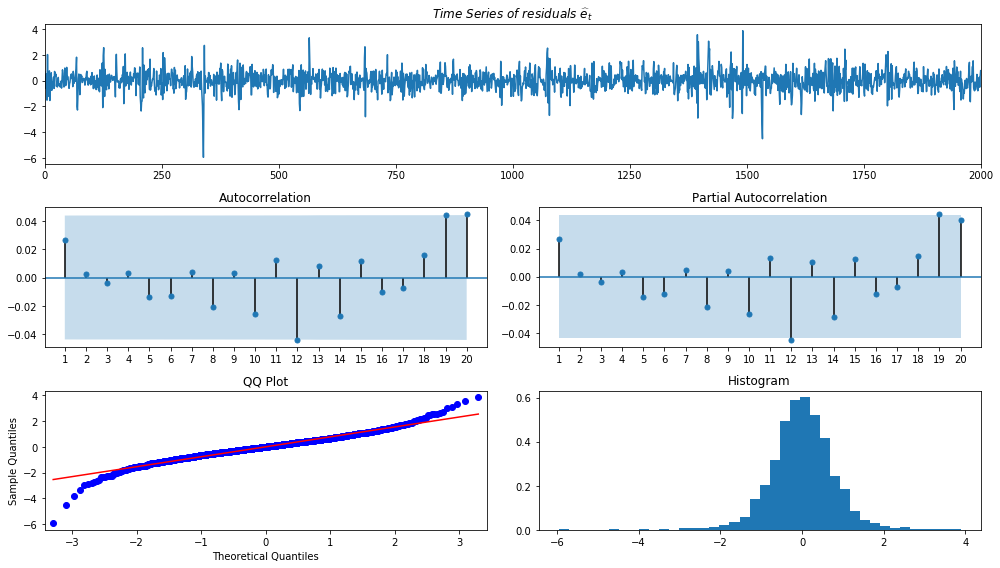In :
tsdiag(resids)Out:
1 2 3 4 5 6 7 8 9 10
Ljung-Box: X-squared 1.436600 1.450969 1.479100 1.495282 1.893443 2.223409 2.258945 3.160586 3.181938 4.539475
Ljung-Box: p-value 0.230690 0.484090 0.687102 0.827477 0.863684 0.898048 0.944130 0.923879 0.956643 0.919747
Box-Pierce: X-squared 1.434448 1.448787 1.476848 1.492982 1.889751 2.218398 2.253774 3.150912 3.172146 4.521547
Box-Pierce: p-value 0.231040 0.484618 0.687624 0.827884 0.864180 0.898557 0.944471 0.924533 0.957077 0.920768

The residuals no not exhibit any significant autocorrelation, but their squared values:

In :
tsdisplay(resids**2, title = "of\ \widehat{e}_t^2")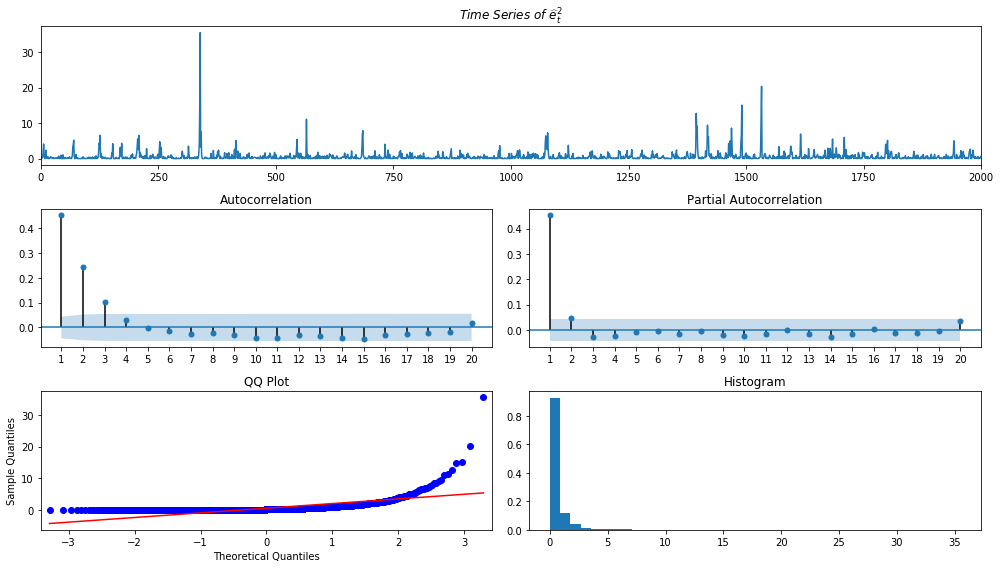exhibit significant autocorrelations. This can again be verified via Ljung-Box test:

In :
tsdiag(resids**2)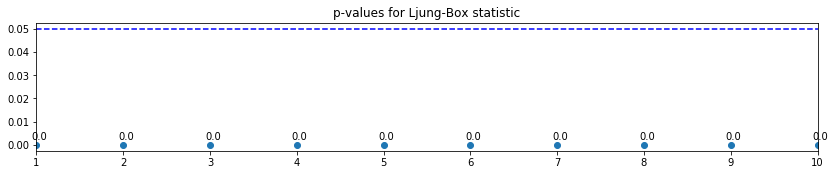Out:
1 2 3 4 5 6 7 8 9 10
Ljung-Box: X-squared 4.118510e+02 5.306804e+02 5.521659e+02 5.537575e+02 5.537961e+02 5.542147e+02 5.554377e+02 5.563951e+02 5.581936e+02 5.616128e+02
Ljung-Box: p-value 1.449457e-91 5.810637e-116 2.357527e-119 1.573765e-118 1.936009e-117 1.742653e-116 9.540885e-116 5.494701e-115 1.948741e-114 2.930530e-114
Box-Pierce: X-squared 4.112339e+02 5.298258e+02 5.512577e+02 5.528445e+02 5.528829e+02 5.532999e+02 5.545174e+02 5.554700e+02 5.572586e+02 5.606574e+02
Box-Pierce: p-value 1.974895e-91 8.908274e-116 3.709561e-119 2.480182e-118 3.048761e-117 2.744299e-116 1.505355e-115 8.682933e-115 3.092030e-114 4.693293e-114

Looking at the PACF plot of the squared residuals, $\widehat{e}_t^2$, we see that the first (and possibly, second?) lag is significantly different from zero, so we would need to specify an $\rm ARCH(1)$ model.

### (3) Specifying a volatility model and performing a joint estimation of the mean and volatility equations¶

We will further assume that the underlying shocks are normal, $w_t \sim \mathcal{N}(0, \sigma^2)$.

• The mean equation
In :
mdl_arch = arch.univariate.ConstantMean(r_t)

• the volatility equation
In :
mdl_arch.volatility = arch.univariate.ARCH(1)

• the shock distribution
In :
mdl_arch.distribution = arch.univariate.Normal()


Now we can estimate the model coefficients:

In :
mdl_arch_fit = mdl_arch.fit()

Iteration:      1,   Func. Count:      5,   Neg. LLF: 2063.9282348989996
Iteration:      2,   Func. Count:     14,   Neg. LLF: 2063.8157793994865
Iteration:      3,   Func. Count:     23,   Neg. LLF: 2063.8022629924844
Iteration:      4,   Func. Count:     30,   Neg. LLF: 2063.6731746227806
Iteration:      5,   Func. Count:     35,   Neg. LLF: 2063.673021823548
Optimization terminated successfully.    (Exit mode 0)
Current function value: 2063.6730218238563
Iterations: 5
Function evaluations: 35


We can examine the model estimates:

In :
print(mdl_arch_fit.summary())

                      Constant Mean - ARCH Model Results
==============================================================================
Dep. Variable:                      y   R-squared:                      -0.000
Mean Model:             Constant Mean   Adj. R-squared:                 -0.000
Vol Model:                       ARCH   Log-Likelihood:               -2063.67
Distribution:                  Normal   AIC:                           4133.35
Method:            Maximum Likelihood   BIC:                           4150.15
No. Observations:                 2000
Date:                Sat, Mar 02 2019   Df Residuals:                     1997
Time:                        23:08:11   Df Model:                            3
Mean Model
========================================================================
coef    std err          t      P>|t|  95.0% Conf. Int.
------------------------------------------------------------------------
mu             1.0141  1.269e-02     79.901      0.000 [  0.989,  1.039]
Volatility Model
========================================================================
coef    std err          t      P>|t|  95.0% Conf. Int.
------------------------------------------------------------------------
omega          0.2746  1.398e-02     19.638  7.283e-86 [  0.247,  0.302]
alpha       0.5270  4.339e-02     12.144  6.160e-34 [  0.442,  0.612]
========================================================================

Covariance estimator: robust


$\begin{cases} r_t &= 1.0141 + \epsilon_t \\ \epsilon_t &= \sigma_t w_t \\ \sigma_t^2 &= 0.2746 + 0.5270 \epsilon^2_{t-1} \end{cases}$

We can look at the coefficients and see that they are similar to the ones we used to generate the series (the true values were $\mu = 1$, $\omega = 0.2$, $\alpha = 0.5$):

In :
print(mdl_arch_fit.params)

mu          1.014073
omega       0.274569
alpha    0.526976
Name: params, dtype: float64


Note that we have estimated an $\rm ARCH$ model, so we have no $\beta$ parameter.

We can also view the $\rm AIC$ and $\rm BIC$ values:

In :
print(pd.DataFrame([mdl_arch_fit.aic, mdl_arch_fit.bic], index = ["AIC", "BIC"], columns = ["ARCH"]).T)

              AIC          BIC
ARCH  4133.346044  4150.148751


• If we wanted to estimate a $\rm GARCH(1, 1)$ model, we would need to specify as follows:
In :
mdl_garch = arch.univariate.ConstantMean(r_t)
mdl_garch.volatility = arch.univariate.GARCH(1, 0, 1)
mdl_garch.distribution = arch.univariate.Normal()


Then. the estimated model is:

In :
mdl_garch_fit = mdl_garch.fit()

Iteration:      1,   Func. Count:      6,   Neg. LLF: 2109.82885006753
Iteration:      2,   Func. Count:     16,   Neg. LLF: 2109.662128949584
Iteration:      3,   Func. Count:     23,   Neg. LLF: 2079.9254071764544
Iteration:      4,   Func. Count:     30,   Neg. LLF: 2074.1544459915485
Iteration:      5,   Func. Count:     37,   Neg. LLF: 2060.8541827726026
Iteration:      6,   Func. Count:     44,   Neg. LLF: 2052.451514595776
Iteration:      7,   Func. Count:     51,   Neg. LLF: 2048.854000342623
Iteration:      8,   Func. Count:     58,   Neg. LLF: 2047.7208398743273
Iteration:      9,   Func. Count:     64,   Neg. LLF: 2047.4171635170721
Iteration:     10,   Func. Count:     70,   Neg. LLF: 2047.3865316526096
Iteration:     11,   Func. Count:     76,   Neg. LLF: 2047.3831321330633
Iteration:     12,   Func. Count:     82,   Neg. LLF: 2047.3830591941799
Optimization terminated successfully.    (Exit mode 0)
Current function value: 2047.3830585248247
Iterations: 12
Function evaluations: 83

In :
print(mdl_garch_fit.summary())

                     Constant Mean - GARCH Model Results
==============================================================================
Dep. Variable:                      y   R-squared:                      -0.000
Mean Model:             Constant Mean   Adj. R-squared:                 -0.000
Vol Model:                      GARCH   Log-Likelihood:               -2047.38
Distribution:                  Normal   AIC:                           4102.77
Method:            Maximum Likelihood   BIC:                           4125.17
No. Observations:                 2000
Date:                Sat, Mar 02 2019   Df Residuals:                     1996
Time:                        23:08:11   Df Model:                            4
Mean Model
========================================================================
coef    std err          t      P>|t|  95.0% Conf. Int.
------------------------------------------------------------------------
mu             1.0155  1.247e-02     81.423      0.000 [  0.991,  1.040]
Volatility Model
========================================================================
coef    std err          t      P>|t|  95.0% Conf. Int.
------------------------------------------------------------------------
omega          0.1809  1.725e-02     10.493  9.336e-26 [  0.147,  0.215]
alpha       0.5118  4.372e-02     11.705  1.199e-31 [  0.426,  0.597]
beta        0.1924  3.523e-02      5.462  4.698e-08 [  0.123,  0.261]
========================================================================

Covariance estimator: robust


$\begin{cases} r_t &= 1.0155 + \epsilon_t \\ \epsilon_t &= \sigma_t w_t \\ \sigma_t^2 &= 0.1809 + 0.5118 \epsilon^2_{t-1} + 0.1924 \sigma^2_{t-1} \end{cases}$

The true values were: $\mu = 1$, $\omega = 0.2$, $\alpha = 0.5$ and $\beta = 0.3$. The $\widehat{\beta}$ estimate is not as close to the true value as $\widehat{\mu}$, $\widehat{\alpha}$ and $\widehat{\omega}$.

Note the $\rm AIC$ and $\rm BIC$

In :
print(pd.DataFrame([mdl_garch_fit.aic, mdl_garch_fit.bic], index = ["AIC", "BIC"], columns = ["GARCH"]).T)

               AIC          BIC
GARCH  4102.766117  4125.169727


Note that the $\rm AIC$ and $\rm BIC$ values are smaller than the ones for the $\rm ARCH$ model, indicating that this is a better model.

• If we wanted to specify an autoregressive process for the mean and estimate, say a $\rm AR(1)-GARCH(1, 1)$ model, we would do the following
In :
mdl_ar_garch = arch.univariate.ARX(r_t, lags = 1)

In :
mdl_ar_garch.volatility = arch.univariate.GARCH(1, 0, 1)

In :
mdl_ar_garch.distribution = arch.univariate.Normal()


Then the estiamted model is:

In :
mdl_ar_garch_fit = mdl_ar_garch.fit()

Iteration:      1,   Func. Count:      7,   Neg. LLF: 2109.5837134396315
Iteration:      2,   Func. Count:     18,   Neg. LLF: 2109.1010104352763
Iteration:      3,   Func. Count:     29,   Neg. LLF: 2108.349432778391
Iteration:      4,   Func. Count:     37,   Neg. LLF: 2078.7188433505657
Iteration:      5,   Func. Count:     45,   Neg. LLF: 2073.0395569273196
Iteration:      6,   Func. Count:     53,   Neg. LLF: 2059.7823795823974
Iteration:      7,   Func. Count:     61,   Neg. LLF: 2051.339040467455
Iteration:      8,   Func. Count:     69,   Neg. LLF: 2047.7834230337362
Iteration:      9,   Func. Count:     77,   Neg. LLF: 2046.6801648986202
Iteration:     10,   Func. Count:     84,   Neg. LLF: 2046.342863757498
Iteration:     11,   Func. Count:     91,   Neg. LLF: 2046.322370518488
Iteration:     12,   Func. Count:     98,   Neg. LLF: 2046.3208415270537
Iteration:     13,   Func. Count:    105,   Neg. LLF: 2046.3207937515638
Optimization terminated successfully.    (Exit mode 0)
Current function value: 2046.320793751422
Iterations: 13
Function evaluations: 105

In :
print(mdl_ar_garch_fit.summary())

                           AR - GARCH Model Results
==============================================================================
Dep. Variable:                      y   R-squared:                      -0.000
Mean Model:                        AR   Adj. R-squared:                 -0.001
Vol Model:                      GARCH   Log-Likelihood:               -2046.32
Distribution:                  Normal   AIC:                           4102.64
Method:            Maximum Likelihood   BIC:                           4130.64
No. Observations:                 1999
Date:                Sat, Mar 02 2019   Df Residuals:                     1994
Time:                        23:08:12   Df Model:                            5
Mean Model
==============================================================================
coef    std err          t      P>|t|       95.0% Conf. Int.
------------------------------------------------------------------------------
Const           1.0214  2.680e-02     38.112      0.000      [  0.969,  1.074]
y       -6.1366e-03  2.429e-02     -0.253      0.801 [-5.374e-02,4.147e-02]
Volatility Model
========================================================================
coef    std err          t      P>|t|  95.0% Conf. Int.
------------------------------------------------------------------------
omega          0.1812  1.737e-02     10.433  1.757e-25 [  0.147,  0.215]
alpha       0.5117  4.377e-02     11.691  1.422e-31 [  0.426,  0.598]
beta        0.1920  3.540e-02      5.424  5.827e-08 [  0.123,  0.261]
========================================================================

Covariance estimator: robust


We can extract the parameters and round them to 4 digits:

In :
np.round(mdl_ar_garch_fit.params, 4)

Out:
Const       1.0214
y       -0.0061
omega       0.1812
alpha    0.5117
beta     0.1920
Name: params, dtype: float64

So, the $AR(1)-GARCH(1,1)$ model is:

$\begin{cases} r_t &= 1.0214 - 0.0061 r_{t-1} + \epsilon_t \\ \epsilon_t &= \sigma_t w_t \\ \sigma_t^2 &= 0.1812 + 0.5117 \epsilon^2_{t-1} + 0.1920 \sigma^2_{t-1} \end{cases}$

Note that the mean and volatility parameters are similar to the $\rm GARCH(1,1)$ model, with an additional $\widehat{\phi} = -0.0061$ autoregression coefficient in the mean equation.

If we look at the $\rm AIC$ and $\rm BIC$ values:

In :
print(pd.DataFrame([mdl_ar_garch_fit.aic, mdl_ar_garch_fit.bic], index = ["AIC", "BIC"], columns = ["AR-GARCH"]).T)

                  AIC          BIC
AR-GARCH  4102.641588  4130.643599


We can compare with the $\rm GARCH(1, 1)$ model

In :
print(pd.DataFrame([mdl_ar_garch_fit.aic - mdl_garch_fit.aic, mdl_ar_garch_fit.bic - mdl_garch_fit.bic], index = ["AIC", "BIC"], columns = ["Difference"]).T)

                AIC       BIC
Difference -0.12453  5.473872


We see that the $\rm AIC$ is slightly lower, but $\rm BIC$ is larger. Furthermore, the increase in $\rm BIC$ is larger than the decrease in $\rm AIC$. Finally, since $\rm BIC$ penalizes model complexity more heavily than $\rm AIC$, we conclude that the $AR(1)-GARCH(1, 1)$ model is not better than the $\rm GARCH(1, 1)$ model.

Finally, if we wanted, we could specify other distributions for the shocks, like Student's $t$ with arch.univariate.StudentsT().

### (4) Model residual checking¶

Finally, we examine the standardized residuals to make sure that there are no more $\rm ARCH$ effects (nor any autocorrelation). The standardized residuals can be computed by dividing the residuals by the conditional volatility.

In :
std_resid = mdl_arch_fit.resid / mdl_arch_fit.conditional_volatility

In :
tsdisplay(std_resid, title = 'of\ ARCH\ standardizez\ residuals')